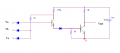# Circuit with transistors and diodes

Joined Feb 9, 2017
13
I need help with the following question:

For the circuit in attach 1, determine the logic function and minimum resistance value of the resistor Rcmin such that the output transistor is in saturation state when it is on.
If the output transistor is charged with capacitor Cp=220nF, determine the fall and rise time of the output voltage.
Given data: βmin=50, β=100, Vcc=5V, Ubet=0.5V, Ubes=0.7V, Ube=Ud=0.6V, Uces=0.2V.

Note: This is not homework, I am practicing for exam.

Attempt:
We consider the static analysis of a circuit (low and high logic level).

Note: I assumed that diodes are idealized (when it is forward biased the voltage is 0.6V, and when it is reverse biased, it is an open branch).

1. Low logic level (Vin=0V)
We don't consider this case because we can never have Vin=0V.
Is this correct?

2. High logic level (Vin=Vcc=5V)
We conclude that at least one input diode is forward biased.
Fourth diode (that is connected to emitter of input voltage) is reverse biased.
Output transistor is on. We need to check if it is in saturation state.
The condition is Ics>Ib/βmin. We can find Ib as Ib=Ubes/5k=0.14mA and Ics as Ics=Uces/Rcmin. This gives that Rcmin>200/7 ?.
Is this correct?

Where to connect capacitor Cp and how to find fall and rise time of Vout?
What is the value of Vout in this case?
How to determine the logic function of this circuit?Last edited by a moderator:

#### WBahn

Joined Mar 31, 2012
25,546
Why can't Vin (at one or more of the inputs) be 0 V?

I'm not quite sure what is meant by "the output transistor is charged with capacitor Cp=220nF". Is this just saying that there is effectively 200 nF of capacitance between Vout and ground?

If Vin is 5V, how can any of the input diodes be forward biased?

To figure out the logic function, either think it through or, to be sure you cover all the bases, write out the truth table. Just by looking at the circuit you should be able to conclude some things about the truth table -- for instance, does it matter how many inputs are low as long as at least one of them is low?

When you are figuring out your rise and fall times, there is a hidden gotcha in the fall-time calculation.

#### MrAl

Joined Jun 17, 2014
7,465
Hi,

It looks like a lot of wording problems in this question.

First, if we have a logic high output then yes one input diode is forward biased, and the input does not have to be 0v although it will be close to that (like 0.2v).

Second, we dont really 'charge' a transistor. It is true that the base gets 'charged' in a way but we dont usually call that "charging a transistor". Before we can calculate any rise or fall times we have to know the correct connections which requires more careful wording of the problem statement. For example the capacitor may be from collector to ground or from collector to +Vcc.

The logic function is the easiest of all here. Just note what it takes on the input to get a low output. You can also look at what it takes to get a high output from certain input states.

Joined Feb 9, 2017
13
@MrAl , Could you explain how to determine the logic function using the truth table?

#### MrAl

Joined Jun 17, 2014
7,465
@MrAl , Could you explain how to determine the logic function using the truth table?
Hi,

Well if you want to do it that way, then you run the inputs at all the possible states.
Because you have three inputs, that means you have 2^3=8 states, in binary from 0 to 7,
So run the three inputs according to the binary count 0 to 7 and note the output state for each combination forming a table.
You can then figure out the logic function from inspection of that table.

#### davideather

Joined Dec 12, 2016
33
the circuit is digital - you are only interested in highs or lows. so consider it for analysis to be 0 or 5 volt. If all the inputs are high than the first transistor (from left to right) is turned on via the 2k resistor. The second transistor is also turned on via the current through the emmiter of t1. Current through t2 shorts the t2 collector to zero. So it has an inverting function.

now consider when one of the inputs are low. this robs base current from t1 stoping it from turning on. That stops t2 turning on and means the output is pulled high by the 6k resistor. All the inputs are the same - if any is low the output will high.

This is a 3 input NAND gate

A B C | O
=====|==
H H H | L
L X X | H
X L X | H
X X L | H

the other bit is horribly worded. I imagine it is 220nf from the base of t2 to gnd but...